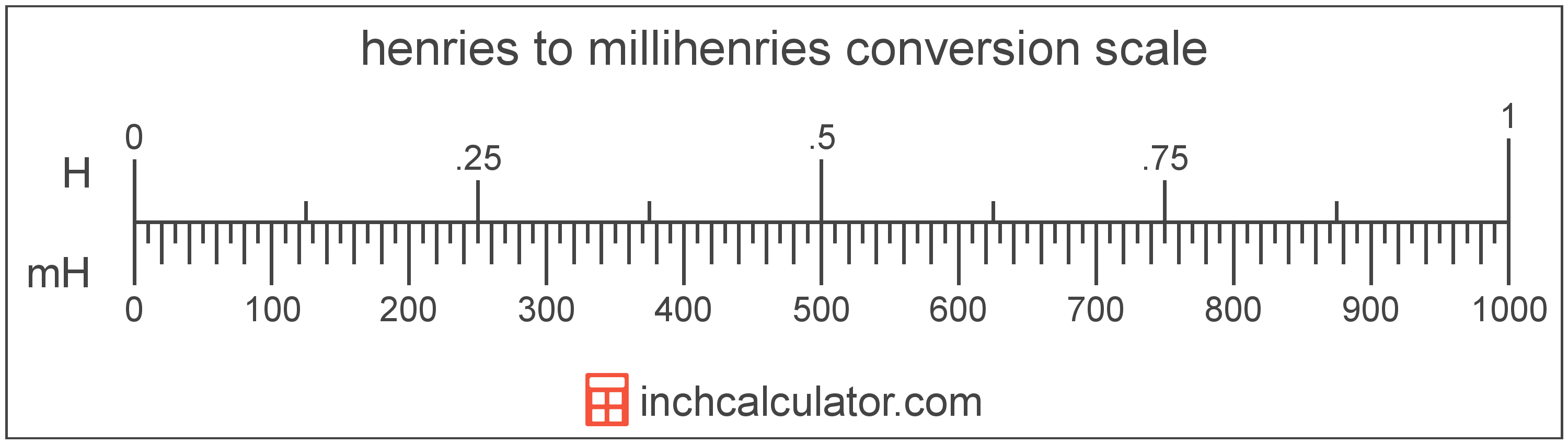# Millihenries to Henries Converter

Enter the electrical inductance in millihenries below to get the value converted to henries.

Results in Henries:1 mH = 0.001 H

Do you want to convert henries to millihenries?

## How to Convert Millihenries to Henries

To convert a measurement in millihenries to a measurement in henries, divide the electrical inductance by the following conversion ratio: 1,000 millihenries/henry.

Since one henry is equal to 1,000 millihenries, you can use this simple formula to convert:

henries = millihenries ÷ 1,000

The electrical inductance in henries is equal to the electrical inductance in millihenries divided by 1,000.

For example, here's how to convert 5,000 millihenries to henries using the formula above.
henries = (5,000 mH ÷ 1,000) = 5 H## What Is a Millihenry?

One millihenry is equal to 1/1,000 of a henry, which is the inductance of a conductor with one volt of electromotive force when the current is increased by one ampere per second.

The millihenry is a multiple of the henry, which is the SI derived unit for electrical inductance. In the metric system, "milli" is the prefix for thousandths, or 10-3. Millihenries can be abbreviated as mH; for example, 1 millihenry can be written as 1 mH.

## What Is a Henry?

One henry is equal to the inductance of a conductor in which there is one volt of electromotive force when the current through the conductor is increased by one ampere per second.

The henry is the SI derived unit for electrical inductance in the metric system. Henries can be abbreviated as H; for example, 1 henry can be written as 1 H.

The National Institute of Standards and Technology recommends using "henries" as the plural form of the unit, however the usage of "henrys" is also common.

## Millihenry to Henry Conversion Table

Table showing various millihenry measurements converted to henries.
Millihenries Henries
1 mH 0.001 H
2 mH 0.002 H
3 mH 0.003 H
4 mH 0.004 H
5 mH 0.005 H
6 mH 0.006 H
7 mH 0.007 H
8 mH 0.008 H
9 mH 0.009 H
10 mH 0.01 H
20 mH 0.02 H
30 mH 0.03 H
40 mH 0.04 H
50 mH 0.05 H
60 mH 0.06 H
70 mH 0.07 H
80 mH 0.08 H
90 mH 0.09 H
100 mH 0.1 H
200 mH 0.2 H
300 mH 0.3 H
400 mH 0.4 H
500 mH 0.5 H
600 mH 0.6 H
700 mH 0.7 H
800 mH 0.8 H
900 mH 0.9 H
1,000 mH 1 H

## References

1. International Bureau of Weights and Measures, The International System of Units, 9th Edition, 2019, https://www.bipm.org/documents/20126/41483022/SI-Brochure-9-EN.pdf
2. Ambler Thompson and Barry N. Taylor, Guide for the Use of the International System of Units (SI), National Institute of Standards and Technology, https://physics.nist.gov/cuu/pdf/sp811.pdf